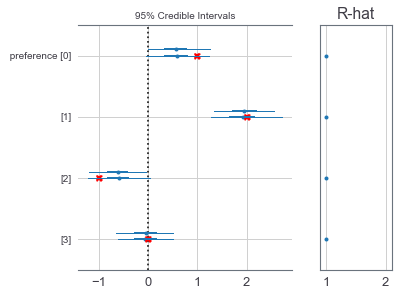# Inferring attribute preferences

Posted on July 11, 2018 • Tagged with pymc3
Download Notebook

In this post, I demonstrate how one can use PyMC3 to infer a person's preferences for several attributes based on the choices they make between sets of items (for which we know the attribute values).

In :
%matplotlib inline
import matplotlib.pyplot as plt
import numpy as np

import warnings
warnings.simplefilter('ignore')  # Don't do this. This is bad.

import pymc3 as pm
import theano.tensor as tt

# Not necessary, but it makes plots look nice
import seaborn as sns
sns.set_context('notebook', font_scale=1.3)
sns.set_palette('tab10')


## Generate a fake choice dataset¶

Imagine we have data from one participant making 200 three-alternative choices. The items are all unique, but they share a set of four features given by $\phi$. We assume that the participant makes choices by noisly maximizing utility given by $U$, and that the utility of each option is a weighted sum of those features. $\alpha$ is an inverse-temperature parameter.

$$U(c) = \theta^\top \phi(c) \\ \Pr(c \mid \mathcal{C}) = \frac {e^{\alpha U(C)}} {\sum_{c' \in \mathcal{C}} e^{\alpha U(c')}}\\$$

In :
# Stimuli
N_FEATURE = 4
N_TRIAL = 200
N_CHOICE = 3
choice_sets = np.random.rand(N_TRIAL, N_CHOICE, N_FEATURE)

# Unknown human parameters
CHOICE_TEMP = 1
PREFERENCE = [1, 2, -1, 0]

def softmax(x, temp=1, np=np):
ex = np.exp((x - x.max(-1, keepdims=True)) / temp)
return ex / ex.sum(-1, keepdims=True)

def fake_data(preference, choice_temp):
utility = np.dot(choice_sets, preference)
p_choice = softmax(utility, temp=choice_temp)
choices = pm.Categorical.dist(p_choice).random()
return choices

choices = fake_data(PREFERENCE, CHOICE_TEMP)


## Inference¶

The model code looks remarkably similar to the code we used to actually generate the data, but instead of specifying the preferences, we create a random variable that we will infer a posterior distribution over.

In :
with pm.Model() as model:
preference = pm.Normal('preference', 0, 10, shape=4)  # somewhat weak prior

# In some cases it makes sense to infer the decision temperature. However,
# in this case, temperature effectively scales the preference, so you can't
# identify both. You can include both though—the model will just find the
# tradeoff that maximizes the prior probability.
# temp = pm.HalfCauchy('temp', 1)  # weak prior
temp = 1

utility = tt.dot(choice_sets, preference)
p_choice = softmax(utility, np=tt)  # use theano instead of numpy for efficiency
pm.Categorical('choices', p_choice, observed=choices)
trace = pm.sample()  # Run MCMC to generate samples from the posterior

Auto-assigning NUTS sampler...
Initializing NUTS using jitter+adapt_diag...
Multiprocess sampling (2 chains in 2 jobs)
NUTS: [preference]
100%|██████████| 1000/1000 [00:02<00:00, 436.96it/s]


## Results¶

We are able to recover the parameters with reasonable accuracy. Note that there are two estimates for each parameter. Each is from a separate MCMC chain. If the two chains make very different predictions, try increasing the number of samples, or use more than two chains e.g. pm.sample(njobs=30) to wash out the bias in each individual chain. If you go this route, you might want to run it on a computer with many CPUs so that the chains can run in parallel.

In :
pm.forestplot(trace, varnames=['preference'])
# Plot the true values with red Xs
plt.sca(plt.gcf().get_axes())
plt.plot(PREFERENCE, -np.arange(N_FEATURE), 'X', c='red');